# Potential theory, mixed boundary value problems of

(diff) ← Older revision | Latest revision (diff) | Newer revision → (diff)

The kind of boundary value problems where the Dirichlet boundary condition (cf. Dirichlet problem) is given at one part of the boundary, and a Neumann-type boundary condition (cf. Neumann problem) is prescribed at the remaining part. Mixed boundary value problems can be encountered in almost any branch of engineering and are among the most difficult to solve. The first solved in the 19th century were the problems of the electrified circular disc and of the spherical cap [a1]. The method used may be classified as a Green's function method, with the main drawback that the Green's function in question was constructed rather than derived. Success of the procedure depended mainly on the researcher's ingenuity and imagination. Not many problems can be solved this way. Various integral transform methods were developed in the first half of the 20th century. Some of the initial results were described in [a7]. One can find in the contemporary literature [a8], [a9] two major methods for solving mixed boundary value problems: the integral transform method, leading to dual integral equations, and the method of dual series equations. Both methods are capable of solving axisymmetric problems, but when one needs to solve a non-axisymmetric problem, the results for each harmonic have to be obtained separately, usually by a very cumbersome process which becomes more and more complicated as the number of harmonics increases. The new general approach systematically presented in [a2] made it possible for the first time to solve non-axisymmetric problems by elementary means, exactly and in closed form. The new method also allows one to analytically treat non-classical domains. A vast variety of shapes has been investigated in [a2], and the accuracy of analytic solutions was found to be surprisingly high.

In order to highlight the difference between the new method and the old one, here is how the problem of a circular disc, charged to a potential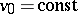, is solved by the dual integral equation method. It is necessary to find a harmonic function, vanishing at infinity, and subjected to the mixed boundary conditions on the plane: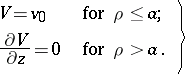(a1)

The solution is presented in the form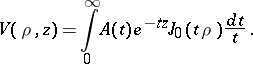(a2)

Here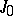is the Bessel function of zero order (cf. Bessel functions), and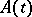is the as yet unknown function, which should be chosen to satisfy (a1). Substitution of the boundary conditions (a1) in (a2) leads to the dual integral equations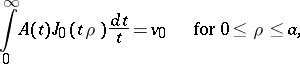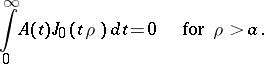By using the discontinuous Weber–Schafheitlin integrals, one can deduce that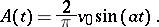The solution (a2) can now be rewritten as(a3)

As one can see, the solution, even to the simplest problem, is not so simple.

The new method is based on the general integral representation for the distance between two points in cylindrical coordinates: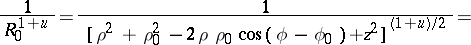(a4)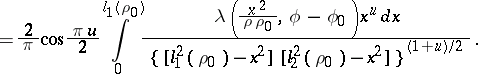Here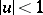, and the following notation was introduced: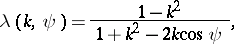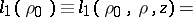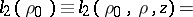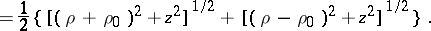The main feature of (a4) is factorization of the parameters related to different points, and also the fact that the angle between their position radii is no longer inside an irrational expression.

The new method can solve general non-axisymmetric problems by elementary means. Consider, for example, the problem of finding a potential function, harmonic in the half-space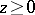, vanishing at infinity and subject to the following boundary conditions on the plane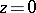: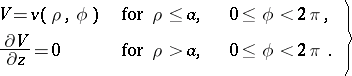(a5)

The problem (a5) can be interpreted as an electrostatic problem of a charged disc, with a non-uniform potential prescribed on its surface, or it can be interpreted as an elastic contact problem of a circular punch pressed against an elastic half-space; other interpretations are also possible. The problem is called interior because the non-zero conditions are prescribed inside the disc. The potential functioncan be represented through the simple-layer potential as follows: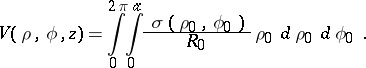(a6)

Here(a7)

Substitution of the boundary condition (a5) in (a6) leads to the governing integral equation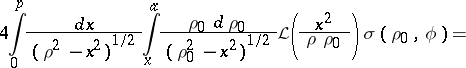(a8)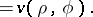Here the operator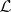is defined byThe main advantage of the new method is that the governing integral equation is presented as a sequence of two Abel-type operators and anoperator. Since the inverse to each of them is known, the exact closed-form solution of equation (a8) is, [a2]:(a9)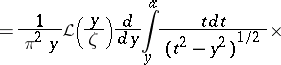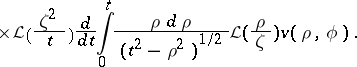The reverse substitution of (a9) in (a6) allows one to express the potential in space in terms of its prescribed value on the disc as follows: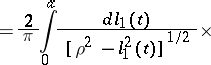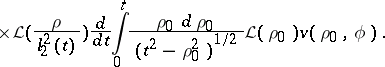In the particular case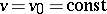, the last expression yields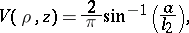which is much simpler than the equivalent solution (a3).

Now consider yet another fundamental interior problem, characterized by the following mixed conditions on the boundary: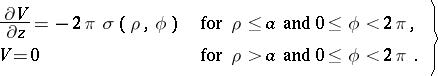(a10)

The problem (a10) can be interpreted as an electrostatic problem of a charged disc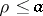inside an infinite grounded diaphragm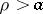. A mathematically similar problem arises in the consideration of a penny-shaped crack subjected to an arbitrary normal pressure. The exact solution to the problem takes the form, [a2]: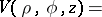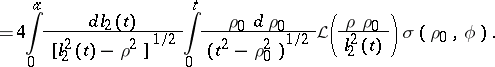The relevant exterior problems can be solved in a similar fashion. The new method was successfully applied to more complicated problems in electromagnetics [a4], acoustics [a3] and diffusion [a6]. Some problems in fluid mechanics and heat transfer are among other possible applications of the new method.

Utilization of the new method in various systems of coordinates allows one to effectively use different geometries. A transition to spherical coordinates was made in [a5]. It is known that transition to toroidal coordinates is also possible. Here are some relevant, yet (1990) unpublished, results.

The distance between two points with toroidal coordinatesandcan be written as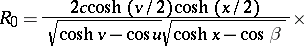(a11)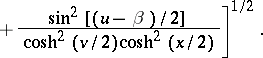Hereis a dimensional parameter. By using previous results one can obtain the following integral representation for the reciprocal of the distance between two points: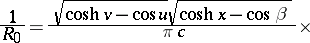(a12)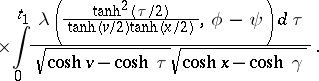Here the following notation was introduced: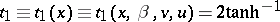The usefulness of the integral representation (a12) can be demonstrated by solving the Dirichlet problem for a spherical cap with the following condition on its surface: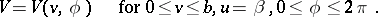(a13)

The as yet unknown potential in space can be represented through a simple-layer distribution:(a14)

Hereis the charge distribution and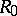is defined by (a11). Substitution of (a12) in (a14) yields, after changing the order of integration,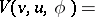(a15)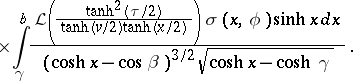Substituting the boundary condition (a13) in (a15) results in the governing integral equation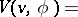(a16)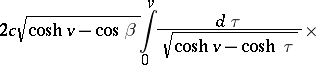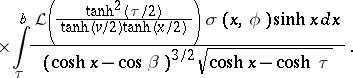The exact closed-form solution of (a16) is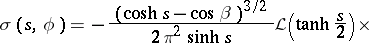(a17)One can now substitute (a17) in (a15) in order to obtain the potential in space through its value on the spherical cap. The following result can be obtained:Here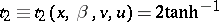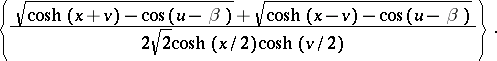The problem of determining the complete set of coordinates in which the new method can be successfully applied is still unsolved.

How to Cite This Entry:
Potential theory, mixed boundary value problems of. Encyclopedia of Mathematics. URL: http://encyclopediaofmath.org/index.php?title=Potential_theory,_mixed_boundary_value_problems_of&oldid=18969
This article was adapted from an original article by V.I. Fabrikant (originator), which appeared in Encyclopedia of Mathematics - ISBN 1402006098. See original article